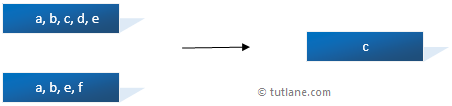# LINQ Except Method

In LINQ, the Except method or operator is useful for returning only the elements from the first collection that are not present in the second collection.

Following is the pictorial representation of the LINQ Except method.As discussed, it returns elements only from the first collection, which do not exist in the second collection.

## Syntax of LINQ Except Method

Following is the syntax of using the LINQ Except method to get elements from the first list which do not exist in the second list.

C# Code

var result = arr1.Except(arr2);

VB.NET Code

Dim result = arr1.Except(arr2)

If you observe the above syntax, we are comparing two lists, “arr1” and “arr2” and getting the elements using the Except method.

## Example of LINQ Except Method

The following is the LINQ Except method example to get elements from the first collection.

C# Code

using System;
using System.Linq;
using System.Collections.Generic;

namespace Linqtutorials
{
class Program
{
static void Main(string[] args)
{
string[] arr1 = { "Suresh", "Rohini", "Praveen", "Sateesh"};
string[] arr2 = { "Madhav", "Sushmitha", "Sateesh", "Praveen" };
var result = arr1.Except(arr2);
foreach (var item in result)
{
Console.WriteLine(item);
}
}
}
}

VB.NET Code

Module Module1

Sub Main()
Dim arr1 As String() = {"Suresh", "Rohini", "Praveen", "Sateesh"}
Dim arr2 As String() = {"Madhav", "Sushmitha", "Sateesh", "Praveen"}
Dim result = arr1.Except(arr2)
For Each item In result
Console.WriteLine(item)
Next
End Sub
End Module

If you observe the above example, we applied the Except method to “arr1” and “arr2” to get elements from the first collection that do not exist in the second collection.

## Result of LINQ Except Method Example

Following is the result of the LINQ Except method example.

Suresh
Rohini

This is how we can use the LINQ Except method to get elements from the first collection that do not exist in the second collection.